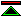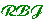# A Short History of Rigour in Mathematics

 Mathematics as Science In the hands of the ancient Greeks mathematics becomes a systematic body of knowledge rather than a collection of practical techniques. Mathematics is established as a deductive science in which the standard of rigorous demonstration is deductive proof. Logic and Axiomatic Method Aristotle provides a codification of logic which remains definitive for two thousand years. The axiomatic method is established and is systematically applied to the mathematics of the classical period by Euclid, whose Elements becomes one of the most influential books in history. Mathematical Logic The next major advances in logic after Aristotle appear in the nineteenth century, in which Boole introduces the propositional (boolean) logic and Frege devises the predicate calculus. This provides the technical basis for the logicisation of mathematics and the transition from informal to formal proof. Russell's paradox and Principia Mathematica Russell's paradox, among others, shakes the foundational advances of Frege, but is quickly resolved. The feasibility of formal development of mathematics is demonstrated by Principia Mathematica. It doesn't catch on. Geometry and Arithmetic The Pythagoreans, were first inclined to regard number theory as more basic than geometry. The discovery of incomensurable ratios presented them with a foundational crisis not fully resolved until the 19th century. Since Greek number theory (which concerns only whole numbers) cannot adequately deal with the magnitudes found in geometry, geometry comes to be considered more fundamental than arithmetic. Analytic Geometry Despite the inadequacies of the available number systems the desire to treat geometry numerically remains. Descartes, by inventing co-ordinate geometry advances an understanding of how geometry can be reduced to number. Calculus on Shifting Sands Mathematics continues to develop as Newton and Leibniz invent the calculus despite weakness in the underlying number system. Berkeley is one of the vocal critics of the soundness of the methods used. The Rigorisation of Analysis Not until the 19th Century do we see the foundational problems resolved by precise definition of the real number system and elimination of the use of infinitesimals from mathematical proofs. Mathematics as Set Theory Cantor's development of set theory together with Frege's advances in logic pave the way for Zermelo's first order axiomatisation of set theory, which provides the foundations for mathematics in the twentieth century.©created 96/8/3 modified 97/1/3